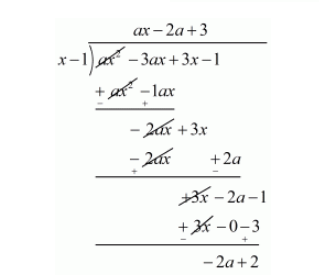# If 1 is a zero of the polynomial p(x) = ax2 − 3(a − 1) x − 1,Question:

If 1 is a zero of the polynomial $p(x)=a x^{2}-3(a-1) x-1$, then find the value of $a$.

Solution:

We know that if $x=\alpha$ is a zero of polynomial then $x-\alpha$ is a factor of $p(x)$

Since 1 is zero of $p(x)$

Therefore, $x-1$ is a factor of $p(x)$

Now, we divide $p(x)=a x^{2}-3(a-1) x-1$ by $x-1$.Now, Remainder $=0$

$-2 a+2=0$

$-2 a=-2$

$a=\frac{-2}{-2}$

$a=1$

Hence, the value of a is 1